# Answer to Question #4565 in Astronomy | Astrophysics for Theresa

Question #4565
Assuming that Earth has a circular orbit with a radius of 1.496 x 10^11 m, what is
a) the average speed (in m/s) o fEarth for one full orbit?
b) the average velocity of Earth for one full orbit?
1
2011-10-11T12:10:23-0400
We are given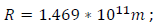a) Average speed defined the length of the path traveled until time t by the time.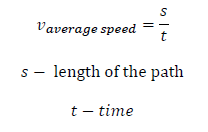length of the path is length of the orbit. Length of circular orbit relates with its radius as: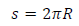One full orbit Earth goes for one year. Hence: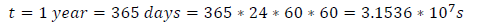Thus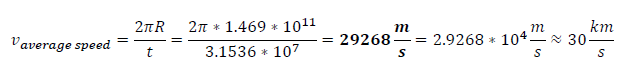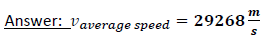Velocity is the measurement of the rate and direction of change in the position of an object. It is a vector physical quantity; both magnitude and direction are required to define it.
The scalar absolute value (magnitude) of velocity is speed.
b) The average velocity v of an object moving through a displacement delta x during a time interval (&Delta;t) is described by the formula: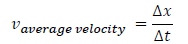After rounding one full orbit Earth is back at its start position, so displacement is zero. Thus: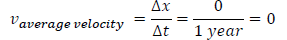Answer: average velocity of Earth for one full orbit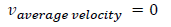Need a fast expert's response?

Submit order

and get a quick answer at the best price

for any assignment or question with DETAILED EXPLANATIONS!